• 作为数学学习辅助性工具，其强大功能为各位生活提供了无数便利，实践出真知，加油吧！
• matlab开发-练习题题。在6个月的练习中，UNE进展是成功
• MATLAB 练习题第1章 MATLAB 简介一、熟悉MATLAB 界面布局和各窗口功能。 二、计算1/3sin(1)e +； 2 3．2/3(8)-。第2章 MATLAB 基本操作一、定义数组、数组维数转换、访问数组元素 1. 用两种方法定义长度为18向量...
MATLAB 练习题第1章 MATLAB 简介一、熟悉MATLAB 的界面布局和各窗口功能。 二、计算1/3sin(1)e +； 2 3．2/3(8)-。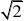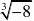第2章 MATLAB 基本操作一、定义数组、数组维数转换、访问数组元素 1. 用两种方法定义长度为18的向量；2．将1中定义的向量变形为36?的矩阵，并访问该矩阵第1、3、5列的元素；3．将1中定义的向量变形为323??的数组，并访问该数组第2行第1列第2页上的元素。二、取整运算，排序1. 运行命令：x = 20*rand(1,12)-10 定义向量x ； 2．将1中定义的向量x 分别按以下方式取整： (1)四舍五入取整； (2)向-∞方向取整； (3)向+∞方向取整； (4)向0方向取整。 3．将2中四舍五入取整后结果进行排序(从小到大)。三、结构体数组、元胞数组定义结构体数组和元胞数组，用来存储以下信息：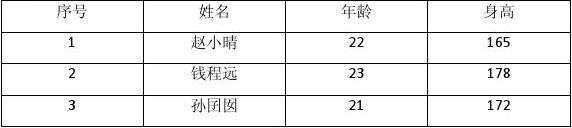第3章 绘图与可视化一、二维图1．现有一组儿童成长记录数据(0-7岁)，包括月龄、身高和体重的观测数据，如下表所示：
展开全文• matlab 练习题和答案 控制系统仿真实验 Matlab 部分实验结果 目 录 实验一 MATLAB基本操 作 1 实验二 Matlab 编 程 5 实验三 Matlab 底层图形控 制 6 实验四 控制系统古典分 析 12 实验五 控制系统现代分 析 15 实验...
• 适用于matlab初学者的练习题，可根据自身情况挑选练习
• 清除Matlab工作空间wordspace变量命令是 B A. clc B. clear C. clf D.delete 2. 清除当前屏幕上显示所有内容但不清除工作空间中数据命令是 A A. clc B. clear C. clf D.delete 3. 用来清除图形命令 C A. ...
• 不是很好 就是一部分学生做课程设计是可可能用得上！嘿嘿
• 第 1 章 MATLAB 概论 1.1 与其他计算机语言相比较 MATLAB 语言突出特点是什么 答 MATLAB 语言有一下特点 1 起点高 2 人机界面适合科技人员 3 强大而简易作图功能 4 智能化程度高 5 功能丰富可扩展性强 1.2 ...
• WORD格式 专业资料整理 1章MATLAB概论 1.1与其他计算机语言相比较 MATLAB 语言突出特点是什么 答MATLAB 语言有一下特点 起点高 人机界面适合科技人员 强大而简易作图功能 智能化程度高 功能丰富可扩展性强 1.2...
• 精品文档 MatLab 练习题 4 - 程 序设计 学号 班级 姓名 注意 : 1, 请按要求作题 , 直接输入答案 , 并保存文件 . 2, 仔细填写 学生信息 . 3, 把此 word 文档文件名更名为你 学号第 * 次 . 上传至 http //1. 例 ...
• ## Matlab基础练习题

千次阅读 2020-03-22 23:25:03
Matlab基础练习题 1、按要求写出实现该功能代码 2、练习矩阵定位函数find使用 3、练习子矩阵赋值、删除等操作 4、练习常用函数使用 5、按要求编写fun函数 叮嘟！这里是小啊呜学习课程资料整理。好记性不如...
Matlab基础练习题1、按要求写出实现该功能的代码2、练习矩阵定位函数find的使用3、练习子矩阵的赋值、删除等操作4、练习常用函数的使用5、按要求编写fun函数
叮嘟！这里是小啊呜的学习课程资料整理。好记性不如烂笔头，今天也是努力进步的一天。一起加油进阶吧！1、按要求写出实现该功能的代码
（1）使用方括号“[ ]”操作符产生一个列向量x，内容为1，2，4，7
X=[1;2;4;7]（2）使用方括号“[ ]”操作符产生一个行向量x，内容为1，2，4，7
X=[1,2,4,7]（3）使用冒号“：”操作符产生一个行向量x，内容为9，7，5，3，1
X=9:-2:1（4）使用方括号“[ ]”操作符产生一个二维数组A，第1行为9，4，5，1；第2行为1，0，4，7
A=[9,4,5,1;1,0,4,7]（5）使用zeros函数产生一个32的二维数组A，使用ones函数产生一个34的二维数组B，将A、B拼接成3*6的二维数组C
A=zeros(3,2)
B=ones(3,4)
C=[A,B]（6）使用rand函数产生一个3*4的二维数组A，使用逻辑1标识的方式寻访A矩阵中大于0.3并且小于0.7的所有元素，赋给B
A=rand(3,4)
B=find(0.3<A<0.7)2、练习矩阵定位函数find的使用
逐句输入并运行以下命令，观察每一步的运行结果，弄明白每一条语句的含义。
clear;
X=[3 2 0; -5 6 1]

indices =find(X>=1)
%%语句1  **返回矩阵中满足条件X>=1的元素的位置**

[row,col] =find(X>=1)
%%语句2   **找出矩阵中满足条件X>=1的元素所在的行和列，并分别存储在row和col中**3、练习子矩阵的赋值、删除等操作
已知：
A =
1     25     7    20
14    23     6    18
12    23    15    16
20    10     5     9
在MATLAB中给A赋值并完成以下操作：

（1）给A中第6个元素赋值为100；
命令语句如下：
A(6)=100（2）给A的第五列赋值[1，2，3，4]；
命令语句如下：
A=[A [1;2;3;4]]（3）将矩阵A第2—4行中第1，3，5列元素赋值给矩阵B；
命令语句如下：
B=A(2:4,1:2:5)（4）求矩阵A的大小（行列数）；
命令语句如下：
size(A)（5）将A赋值给矩阵C，删除C的第一行和第三行并显示；
命令语句如下：
C=A
C(1:2:3,:)=[]（6）将矩阵A重排成5行4列的矩阵（提示：查找reshape函数的帮助）。
命令语句如下：
A = reshape(A,5,4)4、练习常用函数的使用
逐句输入并运行以下命令，观察每一步的运行结果。
（1）
x=[3,2,1,0]
a=length(x)
b=size(x,1)
c=size(x,2)
s=sum(x)

（变量a的结果写在此处）：4
（变量b的结果写在此处）：1
（变量c的结果写在此处）：4
（变量s的结果写在此处）：6小贴士：
size(X,1),返回矩阵X的行数；
size(X,2),返回矩阵X的列数；


（2）
x=[3,2,1,0; 5 6 8 7]
b=size(x,1)
c=size(x,2)
d=size(x,3)      %%MATLAB问题https://bbs.csdn.net/topics/330226812
s=sum(x)
s2=sum(x(:))

（变量b的结果写在此处）：2
（变量c的结果写在此处）：4
（变量d的结果写在此处）：1
（变量s的结果写在此处）：   8 8 9 7
（变量s2的结果写在此处）：32

小贴士：
sum(X)，返回矩阵X每列的和；
sum(X(:))，返回矩阵X所有元素的和；


5、按要求编写fun函数
函数输入参数：一个向量
函数输出参数：该向量中所有大于0的元素的和
功能：求该向量中所有大于0的元素的和
函数调用示例：s=fun([-1, 0, 2, 3, -5, 4])
编写函数文件fun.m内容如下：
function [y]=fun(a)
count=size(a(:));
y=0;
for i=1:count
if a(i)>0
y=y+a(i);
end
endEnding！
更多课程知识学习记录随后再来吧！
就酱，嘎啦！注：
1、我们愈是学习，愈觉得自己的贫乏。 —— 雪莱
2、补充：
【5、按要求编写fun函数】另解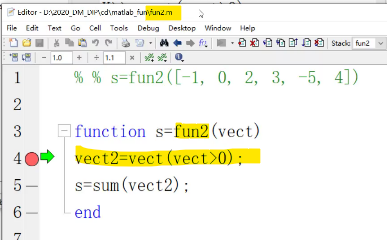展开全文• 精品文档 控制系统仿真实验 Matlab 部分实验结果 精品文档 精品文档 目 录 实验一 MATLAB 基本操作 1 实验二 Matlab 编程 5 实验三 Matlab 底层图形控制 6 实验四 控制系统古典分析 12 实验五 控制系统现代分析 15 ...
• MATLAB练习题(附答案） 《MATLAB语言程序设计》练习题1（2007.4） 一、 填空题 1． 设有程序 A=[1,2,3,4;6,-5,6,3];B=[2,4,5,7];A1=A(:,[1 3 4]);A2=A(1,[2,3]). 将下列命令运行结果填在横线上 size(A)= ;A(1,: )....
• MTA练习题 要求抄题写出操作命令运行结果并根据要求,贴上运行图 求所有根先画图后求解(要求贴图) > solve'exp(x-3,0) > ezl'p(x)-*x2) > o a = 2*ambertw(-/6*3(1/2) 2*lmbertw(1,-1/*3^1/2) -2*abert(163(1/2) 求...
• 光靠跟着教学视频操作是不够，在课后我们需要做一些练习来巩固，所以我会找一些习题来和大家一起做????
光靠跟着教学视频操作是不够的，在课后我们需要做一些练习来巩固，所以我会找一些习题来和大家一起做😄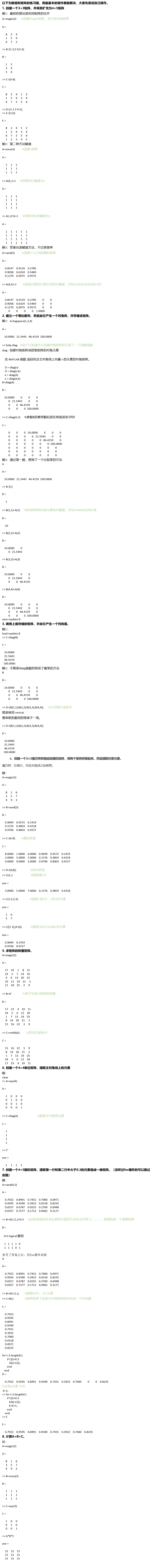展开全文• 练习：讨论平面3自由度3R机器人姿态逆运动解（参考练习题二中模型和参数）。已知固定长度参数：L1 = 4; L2 = 3和L3 = 2 (m).a)用手推导，求这个机器人姿态逆运动学解析解。b）编写二一个matlab程序求解平面3R...
练习：讨论平面3自由度3R机器人的姿态逆运动解（参考练习题二中的模型和参数）。已知固定长度参数：L1 = 4; L2 = 3和L3 = 2 (m).a)用手推导，求这个机器人的姿态逆运动学解析解。b）编写二一个matlab程序求解平面3R机器人的全部姿态逆运动学解，并将每一组的结果代入姿态正运动学matlab程序中验证所有结果。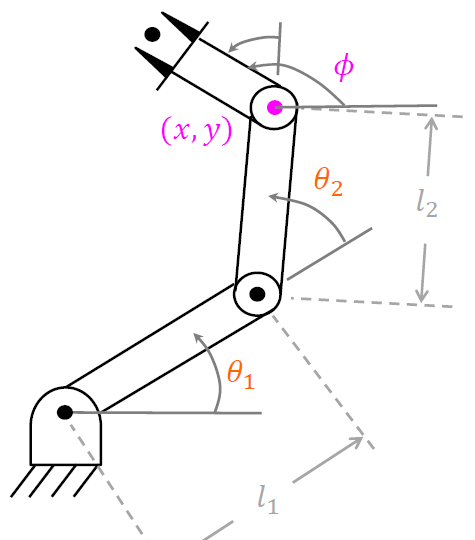风一扬：机器人学导论—matlab练习（二）​zhuanlan.zhihu.com解：3R机器人的逆运动学解在课本中有三种解法。在这我将采用代数方法求解。由练习题（二）我们可知3R机器人的末端相对于基坐标的姿态矩阵如下：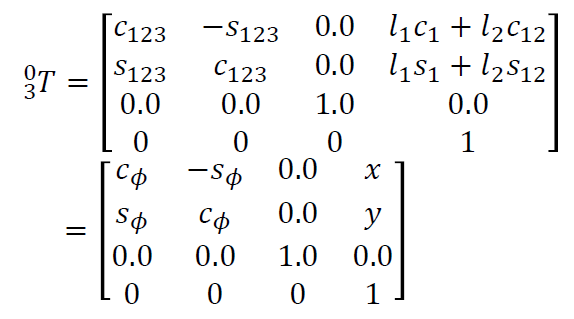（注：）首先求,将x于y进行平方和得：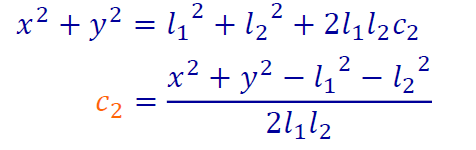由此我们可求出,利用反余弦公式可以得到两个解。将求得得带入x、y得：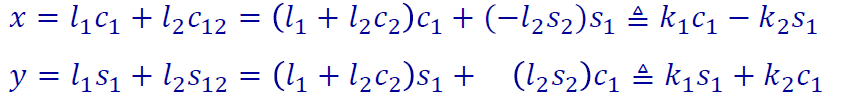进行变换得：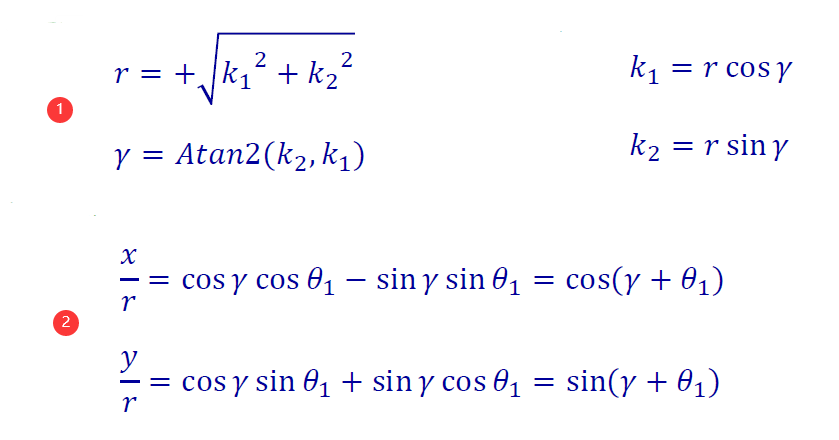从而我们可以解出,当选择不同时，求出得也不相同。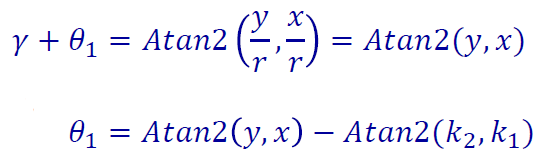最后我们可以通过三个选择角度之和等于，从而求解出。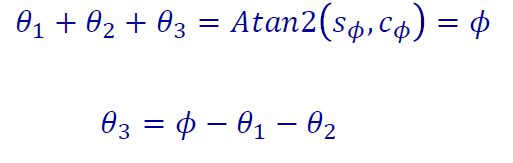除了代数法之外，我们还可以利用几何法和数值法来求解。b)根据上面代数法的计算过程，编写matlab程序求解例题矩阵，并最后运用正运动学验证结果。%定义末端坐标相对于基坐标得齐次矩阵
T0H =  [1 0 0 9
0 1 0 0
0 0 1 0
0 0 0 1];
% T0H =  [ 0.5   -0.866  0  7.5373
%         0.866    0.5   0  3.9266
%           0       0    1     0
%           0       0    0     1  ];
T3H = transl(2,0,0);
%过得计算得齐次矩阵
T = T0H*inv(T3H);
%定义相关参数
X = T(1,4); Y = T(2,4);
L1 = 4; L2 = 3; L3 = 2;
%开始求解角度；
C2 = (X^2+Y^2 -L1^2 -L2^2)/(2*L1*L2);
theta2 = acosd(C2);
%在0°和180°时只有一个解，其他位置有两个解
if theta2 == 0
else
theta2 = [theta2; -theta2];
end
K1 = L1 + L2*cosd(theta2);
K2 = L2*sind(theta2);
r = sqrt(K1.^2+K2.^2);
theta1 = atan2(Y./r,X./r) - atan2(K2,K1);
theta3 = rad2deg(atan2(T(2,1),T(1,1))) - theta1 - theta2;
theta = [theta1 theta2 theta3]

% 定义各个连杆，默认为转动关节
%           theta      d        a     alpha
L(1)=Link([  0         0        0       0],'modified'); L(1).qlim=[-pi,pi];
L(2)=Link([  0         0        4       0],'modified'); L(2).qlim=[-pi,pi];
L(3)=Link([  0         0        3       0],'modified'); L(3).qlim=[-pi,pi];
L(4)=Link([  0         0        2       0  1],'modified');%工具坐标{T}
Robot3 = SerialLink(L,'name','R3');
%验证矩阵
T03 = Robot3.fkine([theta(1,:),0],'deg')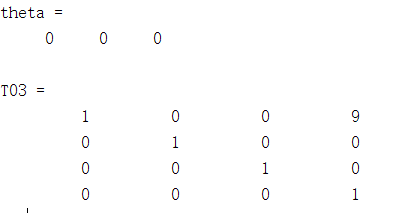例（1）运算结果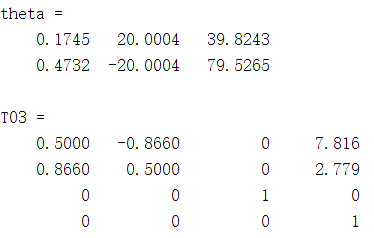例（2）运算结果至此，计算结束。不过在例（2）验算结果与原矩阵有一定误差。（暂时不知道具体原因)
展开全文• 很好的matlab练习题。帮助初学者熟悉matlab软件，是我们学校数学实验题。
• Mat l ab 上机 练习 题及 答案 Matlab Matlab上机练习题及答案 I=A*B J=A/B K=A\B 5 2 4 1 矩阵Y= 0 15 1 给出元素1 45 100 23 21 47 3 全下标和单下标并用函数练习全下标 3利用函数产生3*4阶单位矩阵和全部元素 ...
• <p style="text-align:center"><img alt="" height="710" src="https://img-ask.csdnimg.cn/upload/1611384722586.png" width="736" /></p> <p style="text-align:center"><img alt="" height="662" src...    </p>
• Matlab 基础练习题 常量变量表达式 1MATLAB中下面哪些变量名是合法的 A_num Bnum_ Cnum- D-num 2在 MATLAB中要给出一个复数 z 的模应该使用函 数 Amod(z) Babs(z) Cdouble(z) Dangle(z) 3下面属于 MATLAB的预定义...
• Matlab 上机练习题及答案 1 0 6 8 9 4 0 12.3 4 创建矩阵 a= ,取出其前两 0 0 5.1 2 5 2 4 0 23 0 7 0 15 1 1 矩阵 Y= 给出元素 1 全下标 列构成矩阵 b取出前两行构成矩阵 c 转置矩阵 b 45 100 23 构成矩阵 d ...
• 利用matlab的UI组件完成绘制三角形并计算周长面积，要求：交互式输入三角形交点坐标，其周长和面积的数值通过计算赋值。
• ) 图形，并计算它们所围成立体Ω图形体积。 在这里插入代码片 2、计算积分∬????^???? / ????^???? ???????????????? ，其中积分区域D为由直线???? = ????, ???? = ????和双曲线???????? = ????所围成闭...
• MATLAB数学实验 练习题(附答案) 4.作yoz面上曲线z=sin(y)+1绕z轴旋转所得到图形 5.作xoy面上曲线(x-a)2+y2=r2绕z轴旋转所得到图形(0,取r=1,a=2. 6. 作x2/2-y2/3=2z图形. 7. 作x2/2+y2/3-z2=1图形. %1输入一...
• 4.作yoz面上曲线z=sin(y)+1绕z轴旋转所得到图形%1输入一个字符若输入一个大写字母则输出其对应小写字母若输入一个小写字母则输出其对应大写字母;若为其它字符则原样输出 clear all,clc; ch=input'请输入一个...
• 第 1 章 MATLAB 基础 1.4 MATLAB 操作桌面有几个窗口如何使某个窗口脱离桌面成为独立窗口又如何将脱离出去窗 口重新放置到桌面上 答在 MATLAB 操作桌面上有五个窗口 在每个窗口右上角有两个小按钮 一个是关闭...
• WORD格式 专业资料整理 1章MATLAB基础 1.4MATLAB 操作桌面有几个窗口如何使某个窗口脱离桌面成为独立窗口又如何将脱离出去窗 口重新放置到桌面上 答在MATLAB 操作桌面上有五个窗口 在每个窗口右上角有两个小按钮...
• 关于matlab的ppt和相关内容Matlab教程ppt及练习
• 文章目录1、按要求写出实现该功能代码2、练习矩阵定位函数find使用3、练习子矩阵赋值、删除等操作4、练习常用函数使用：5、按要求编写fun函数： 1、按要求写出实现该功能代码 （1）使用方括号“[ ]”操作...python c++ 数据结构 java
• 学资学习网 学资学习网 精品文档 第 1 章 MATLAB 基础 MATLAB 操作桌面有几个窗口如何使某个窗口脱离桌面成为独立窗口又如何将脱离出 去窗口重新放置到桌面上 答在 MATLAB 操作桌面上有五个窗口在每个窗口右上角......

# matlab的习题练习matlab 订阅